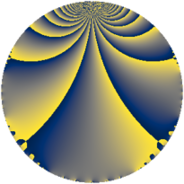# Properties

 Label 6720.2.a.cqLevel $6720$ Weight $2$ Character orbit 6720.a Self dual yes Analytic conductor $53.659$ Analytic rank $0$ Dimension $2$ CM no Inner twists $1$

# Related objects

## Newspace parameters

 Level: $$N$$ $$=$$ $$6720 = 2^{6} \cdot 3 \cdot 5 \cdot 7$$ Weight: $$k$$ $$=$$ $$2$$ Character orbit: $$[\chi]$$ $$=$$ 6720.a (trivial)

## Newform invariants

 Self dual: yes Analytic conductor: $$53.6594701583$$ Analytic rank: $$0$$ Dimension: $$2$$ Coefficient field: $$\Q(\sqrt{3})$$ Defining polynomial: $$x^{2} - 3$$ Coefficient ring: $$\Z[a_1, \ldots, a_{11}]$$ Coefficient ring index: $$2$$ Twist minimal: no (minimal twist has level 3360) Fricke sign: $$-1$$ Sato-Tate group: $\mathrm{SU}(2)$

## $q$-expansion

Coefficients of the $$q$$-expansion are expressed in terms of $$\beta = 2\sqrt{3}$$. We also show the integral $$q$$-expansion of the trace form.

 $$f(q)$$ $$=$$ $$q - q^{3} + q^{5} - q^{7} + q^{9} +O(q^{10})$$ $$q - q^{3} + q^{5} - q^{7} + q^{9} + ( -2 + \beta ) q^{11} + \beta q^{13} - q^{15} + 2 q^{17} + ( -2 + \beta ) q^{19} + q^{21} + 2 \beta q^{23} + q^{25} - q^{27} + 2 q^{29} + ( -2 + \beta ) q^{31} + ( 2 - \beta ) q^{33} - q^{35} -2 q^{37} -\beta q^{39} + ( 2 + 2 \beta ) q^{41} -4 q^{43} + q^{45} + ( 4 - 2 \beta ) q^{47} + q^{49} -2 q^{51} + ( 4 + \beta ) q^{53} + ( -2 + \beta ) q^{55} + ( 2 - \beta ) q^{57} -4 \beta q^{59} + ( -2 - 2 \beta ) q^{61} - q^{63} + \beta q^{65} -2 \beta q^{67} -2 \beta q^{69} + ( 6 - 3 \beta ) q^{71} + ( 4 + \beta ) q^{73} - q^{75} + ( 2 - \beta ) q^{77} + 4 q^{79} + q^{81} + ( -8 + 2 \beta ) q^{83} + 2 q^{85} -2 q^{87} + ( -6 + 2 \beta ) q^{89} -\beta q^{91} + ( 2 - \beta ) q^{93} + ( -2 + \beta ) q^{95} + ( 16 - \beta ) q^{97} + ( -2 + \beta ) q^{99} +O(q^{100})$$ $$\operatorname{Tr}(f)(q)$$ $$=$$ $$2q - 2q^{3} + 2q^{5} - 2q^{7} + 2q^{9} + O(q^{10})$$ $$2q - 2q^{3} + 2q^{5} - 2q^{7} + 2q^{9} - 4q^{11} - 2q^{15} + 4q^{17} - 4q^{19} + 2q^{21} + 2q^{25} - 2q^{27} + 4q^{29} - 4q^{31} + 4q^{33} - 2q^{35} - 4q^{37} + 4q^{41} - 8q^{43} + 2q^{45} + 8q^{47} + 2q^{49} - 4q^{51} + 8q^{53} - 4q^{55} + 4q^{57} - 4q^{61} - 2q^{63} + 12q^{71} + 8q^{73} - 2q^{75} + 4q^{77} + 8q^{79} + 2q^{81} - 16q^{83} + 4q^{85} - 4q^{87} - 12q^{89} + 4q^{93} - 4q^{95} + 32q^{97} - 4q^{99} + O(q^{100})$$

## Embeddings

For each embedding $$\iota_m$$ of the coefficient field, the values $$\iota_m(a_n)$$ are shown below.

For more information on an embedded modular form you can click on its label.

Label $$\iota_m(\nu)$$ $$a_{2}$$ $$a_{3}$$ $$a_{4}$$ $$a_{5}$$ $$a_{6}$$ $$a_{7}$$ $$a_{8}$$ $$a_{9}$$ $$a_{10}$$
1.1
 −1.73205 1.73205
0 −1.00000 0 1.00000 0 −1.00000 0 1.00000 0
1.2 0 −1.00000 0 1.00000 0 −1.00000 0 1.00000 0
 $$n$$: e.g. 2-40 or 990-1000 Significant digits: Format: Complex embeddings Normalized embeddings Satake parameters Satake angles

## Atkin-Lehner signs

$$p$$ Sign
$$2$$ $$1$$
$$3$$ $$1$$
$$5$$ $$-1$$
$$7$$ $$1$$

## Inner twists

This newform does not admit any (nontrivial) inner twists.

## Twists

By twisting character orbit
Char Parity Ord Mult Type Twist Min Dim
1.a even 1 1 trivial 6720.2.a.cq 2
4.b odd 2 1 6720.2.a.cz 2
8.b even 2 1 3360.2.a.bf yes 2
8.d odd 2 1 3360.2.a.bb 2

By twisted newform orbit
Twist Min Dim Char Parity Ord Mult Type
3360.2.a.bb 2 8.d odd 2 1
3360.2.a.bf yes 2 8.b even 2 1
6720.2.a.cq 2 1.a even 1 1 trivial
6720.2.a.cz 2 4.b odd 2 1

## Hecke kernels

This newform subspace can be constructed as the intersection of the kernels of the following linear operators acting on $$S_{2}^{\mathrm{new}}(\Gamma_0(6720))$$:

 $$T_{11}^{2} + 4 T_{11} - 8$$ $$T_{13}^{2} - 12$$ $$T_{17} - 2$$ $$T_{19}^{2} + 4 T_{19} - 8$$ $$T_{23}^{2} - 48$$ $$T_{29} - 2$$ $$T_{31}^{2} + 4 T_{31} - 8$$

## Hecke characteristic polynomials

$p$ $F_p(T)$
$2$ $$T^{2}$$
$3$ $$( 1 + T )^{2}$$
$5$ $$( -1 + T )^{2}$$
$7$ $$( 1 + T )^{2}$$
$11$ $$-8 + 4 T + T^{2}$$
$13$ $$-12 + T^{2}$$
$17$ $$( -2 + T )^{2}$$
$19$ $$-8 + 4 T + T^{2}$$
$23$ $$-48 + T^{2}$$
$29$ $$( -2 + T )^{2}$$
$31$ $$-8 + 4 T + T^{2}$$
$37$ $$( 2 + T )^{2}$$
$41$ $$-44 - 4 T + T^{2}$$
$43$ $$( 4 + T )^{2}$$
$47$ $$-32 - 8 T + T^{2}$$
$53$ $$4 - 8 T + T^{2}$$
$59$ $$-192 + T^{2}$$
$61$ $$-44 + 4 T + T^{2}$$
$67$ $$-48 + T^{2}$$
$71$ $$-72 - 12 T + T^{2}$$
$73$ $$4 - 8 T + T^{2}$$
$79$ $$( -4 + T )^{2}$$
$83$ $$16 + 16 T + T^{2}$$
$89$ $$-12 + 12 T + T^{2}$$
$97$ $$244 - 32 T + T^{2}$$